# Prolongation of solutions of differential equations

The property of solutions of ordinary differential equations to be extendible to a larger interval of the independent variable. Let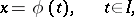(1)

be a solution of the system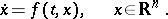(2)

A solution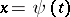,, is called a prolongation of the solution (1) ifandfor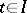.

Suppose that the function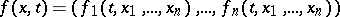is defined in a domain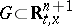and suppose. The solution (1) is called indefinitely extendible (indefinitely extendible forwards (to the right), indefinitely extendible backward (to the left)) if a prolongation of it exists defined on the axis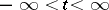(respectively, on the semi-axis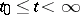, on the semi-axis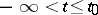). The solution (1) is called extendible forwards (to the right) up to the boundaryofif a prolongation,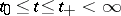, of it exists with the following property: For any compact setthere is a value,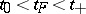, such that the point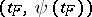does not belong to. Extendibility backward (to the left) up to the boundaryis defined analogously. A solution that cannot be extended is called non-extendible.

If the functionis continuous in, then every solution (1) of (2) can be either extended forwards (backward) or indefinitely or up to the boundary. In other words, every solution of (2) can be extended to a non-extendible solution. If the partial derivatives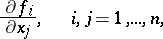(3)

are continuous in, then such a prolongation is unique.

An interval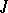is called a maximal interval of existence of a solution of (2) if the solution cannot be extended to a larger interval. For any solution of a linear systemwith coefficients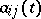and right-hand sides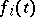,, that are continuous on an interval, the maximal interval of existence of a solution coincides with. For solutions of a non-linear system the maximal intervals of existence may differ for different solutions, and determining them is a difficult task. E.g. for the solution to the Cauchy problem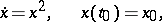one has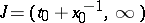if,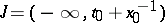if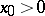, and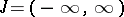if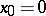.

A sufficient condition under which one can indicate the maximal interval of existence of a solution is, e.g., Wintner's theorem: Suppose that the function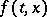is continuous for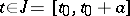,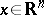, and that it satisfies in this domain the estimatewhereis a function continuous for,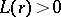and for some,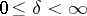,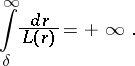Then every solution of (2) exists on the whole of.

This theorem also holds for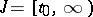. Sufficient conditions for indefinite extendibility of a solution are of great interest. E.g., ifand its partial derivatives (3) are continuous for,, and if for these values ofthe estimates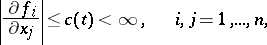hold, then the solution of (2) with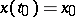exists for, for any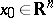.

Consider the Cauchy problem(4)

for an autonomous system, whereis continuously differentiable in a domain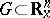. If, asgrows, the phase trajectory of the solutionof (4) remains in a compact subset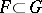, then this solution can be extended to the semi-axis.